# 2 1 Linear Functions as Mathematical Models Unit

• Slides: 11
Download presentation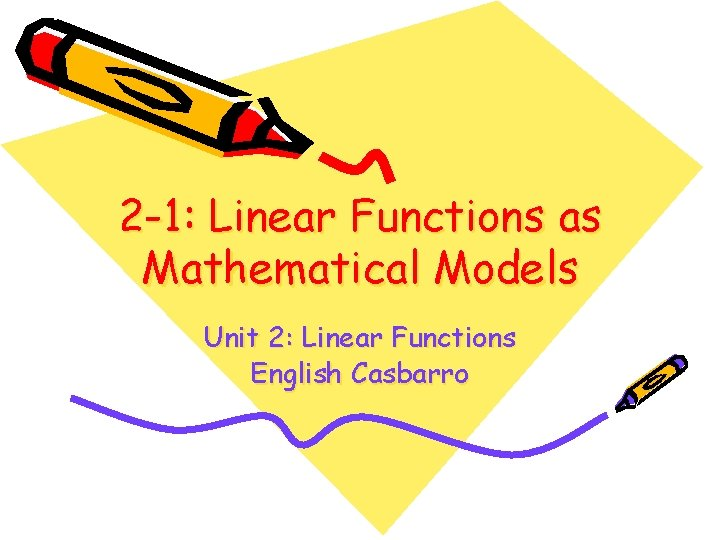2 -1: Linear Functions as Mathematical Models Unit 2: Linear Functions English CasbarroTo solve mathematical models o o o Use the information to write a particular equation (specific to the model) Use the equation to predict values for both variables (depending on the information given) Summarize what the slope and the yintercept tell you about the real-world situation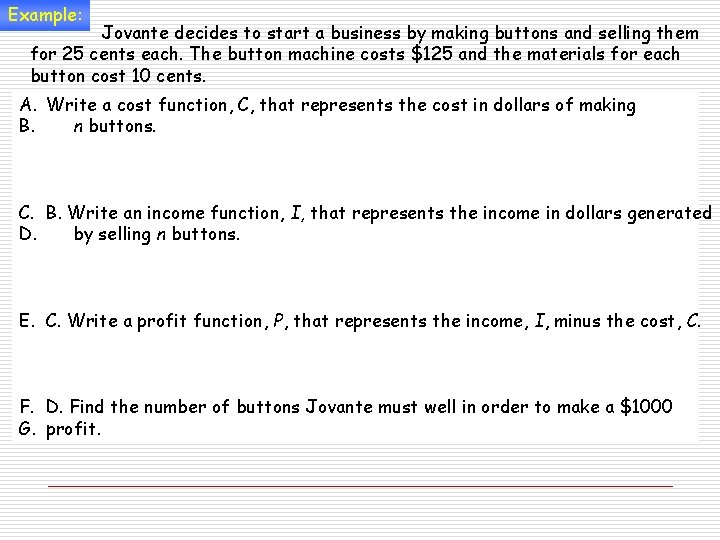Example: Jovante decides to start a business by making buttons and selling them for 25 cents each. The button machine costs \$125 and the materials for each button cost 10 cents. A. Write a cost function, C, that represents the cost in dollars of making B. n buttons. C. B. Write an income function, I, that represents the income in dollars generated D. by selling n buttons. E. C. Write a profit function, P, that represents the income, I, minus the cost, C. F. D. Find the number of buttons Jovante must well in order to make a \$1000 G. profit.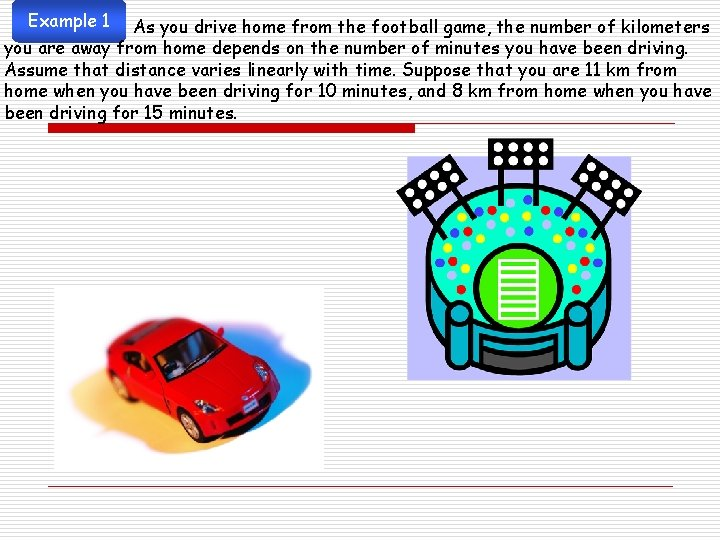Example 1 As you drive home from the football game, the number of kilometers you are away from home depends on the number of minutes you have been driving. Assume that distance varies linearly with time. Suppose that you are 11 km from home when you have been driving for 10 minutes, and 8 km from home when you have been driving for 15 minutes.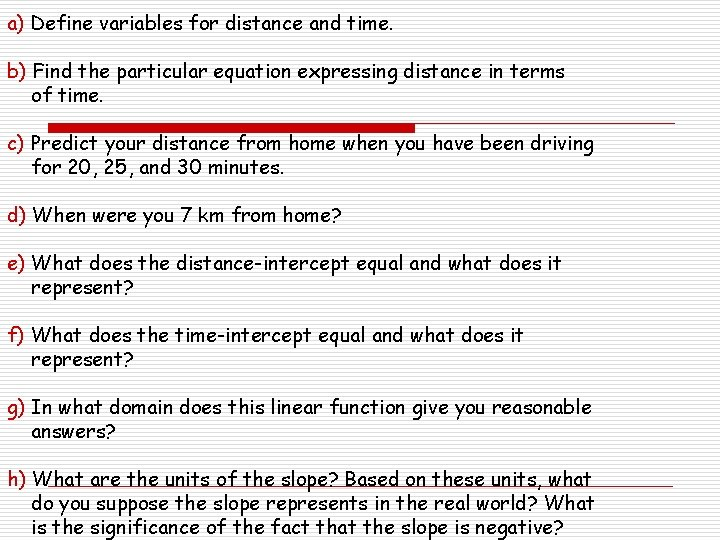a) Define variables for distance and time. b) Find the particular equation expressing distance in terms of time. c) Predict your distance from home when you have been driving for 20, 25, and 30 minutes. d) When were you 7 km from home? e) What does the distance-intercept equal and what does it represent? f) What does the time-intercept equal and what does it represent? g) In what domain does this linear function give you reasonable answers? h) What are the units of the slope? Based on these units, what do you suppose the slope represents in the real world? What is the significance of the fact that the slope is negative?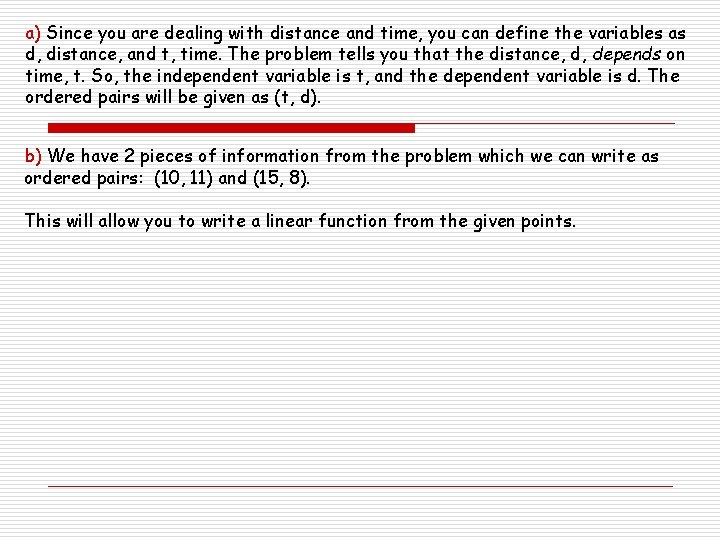a) Since you are dealing with distance and time, you can define the variables as d, distance, and t, time. The problem tells you that the distance, d, depends on time, t. So, the independent variable is t, and the dependent variable is d. The ordered pairs will be given as (t, d). b) We have 2 pieces of information from the problem which we can write as ordered pairs: (10, 11) and (15, 8). This will allow you to write a linear function from the given points.c) Now that we have an equation, you can substitute the values for time into the equation: t = 20 t = 25 t = 30 Note that substituting 30 for t givers a negative value of distance. Since you would not drive past home, the domain of the function should stop before t reaches 30.d) You would substitute distance values into the equation to find time. To predict the time when you are 7 km from home: e) The d-intercept is 17, the value of d when t = 0. When t = 0, you are just leaving for home. Therefore, it must be 17 km between the stadium and home.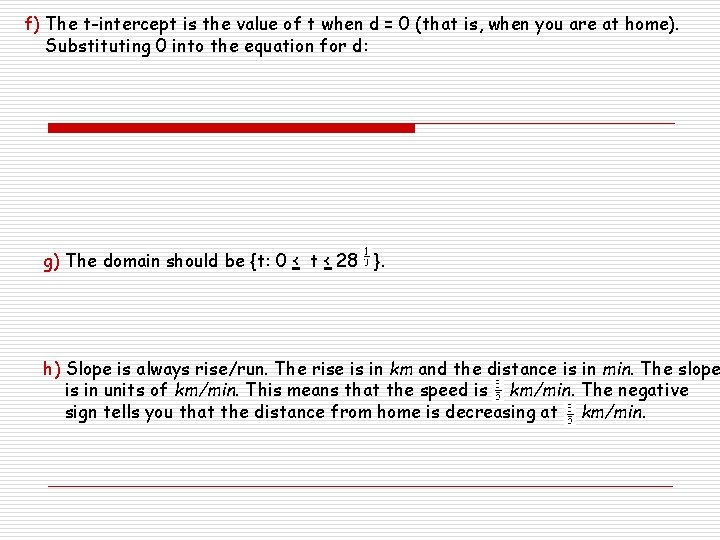f) The t-intercept is the value of t when d = 0 (that is, when you are at home). Substituting 0 into the equation for d: g) The domain should be {t: 0 < t < 28 }. h) Slope is always rise/run. The rise is in km and the distance is in min. The slope is in units of km/min. This means that the speed is km/min. The negative sign tells you that the distance from home is decreasing at km/min.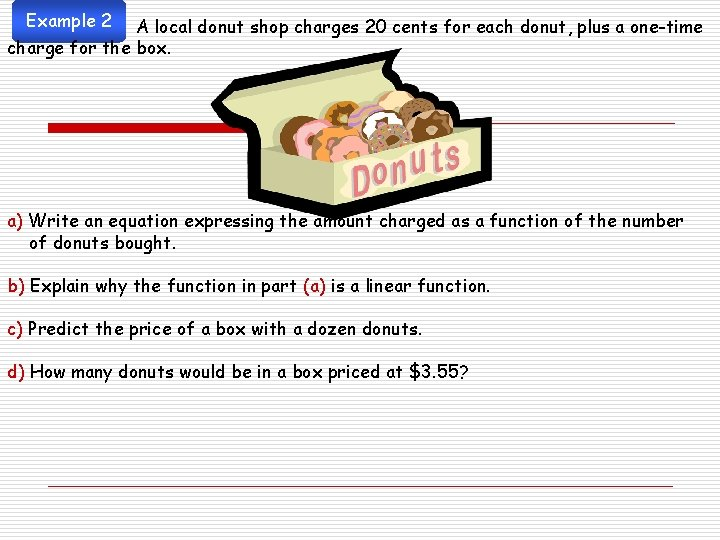Example 2 A local donut shop charges 20 cents for each donut, plus a one-time charge for the box. a) Write an equation expressing the amount charged as a function of the number of donuts bought. b) Explain why the function in part (a) is a linear function. c) Predict the price of a box with a dozen donuts. d) How many donuts would be in a box priced at \$3. 55?1. a) Let d = number of donuts and p = price. The price depends on the number of 2. donuts, so the independent variable is the number of donuts (d, p) 3. The slope is something that repeats again and again. The one-time charge is th 4. price-intercept (where the number of donuts = 0). 5. 6. So, the equation is: p = 20 d + 15 7. b) The equation is linear because the equation has the form p equals a linear 8. expression in the variable d. c) d = 12: p = 2(12) + 15 = 240 + 15 = 255, so the price of 12 donuts is \$2. 55, d) P = 355: 355 = 20 d + 15 340 = 20 d 17 = d, so you would get 17 donuts for \$3. 55.